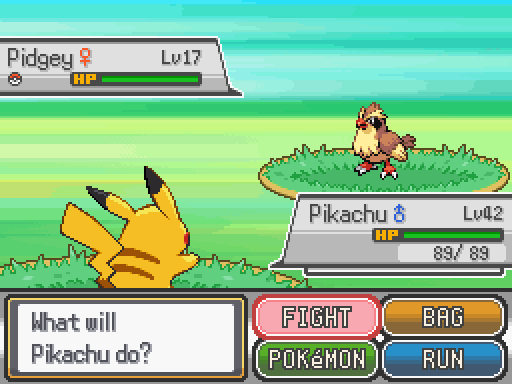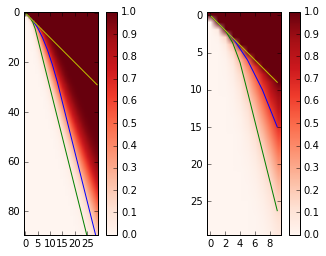Damaging move or status move: that is the questionIntroduction

In all Pokemon’s main series (i.e., from Red/Green/Blue/Yellow to Sun/Moon), at the very beginning of the gameplay, once the player gets his very first Pokemon (e.g., Pikachu), he is invited to battle against his rival (an AI-controlled NPC), who also acquires his first Pokemon (e.g., Eevee). The result of this battle is not critical, but the player can get a bonus if he wins the battle.

In this battle, the player’s Pokemon has a damaging move and a status move. The former deals damage, while the latter changes the statistics (aka stats) of the Pokemon (e.g., raise self’s attack/defense/evasion, reduce the opponent’s attack/defense/accuracy). Meanwhile, the rival’s Pokemon has similar moves. A natural question coming into every player’s mind is whether it is better to spam the damaging move or to look for some smarter tactic. This article uses the optimal stopping theory to answer this classic question.

Solution

If the status moves of both Pokemon cancel each other, it will be useless to use the status move: If the player’s optimal tactic is to use the status move, then the rival’s optimal tactic will surely be to use his status move to cancel the benefit generated by the player’s status move. Luckily, the rival’s AI is not very intelligent, and it is thus reasonable to suppose that the rival spams the damaging move without using the status move. If we can beat the rival’s damaging move spam, then we are likely to beat many of the rival’s playing styles. Consequently, the original question is transformed to another one: encountering such a rival, whether the player can win via the status move if he cannot win by simply spamming the damaging move. The answer is yes: the status move does help in winning the battle.

Let $P$ be the probability of the player’s winning the battle, which is a function of both Pokemon’s statistics $(S_1, S_2)$ and the player’s tactic $A$. The task of the player is to maximize the following optimization problem [ \max_{A \in \mathcal{A}} P(S_1, S_2, A) , ] where $\mathcal{A} = \{\text{All tactics}\}$.

Reason tells us that there is no point in using the status move once one has started using the damaging move. Otherwise, one may just reverse the order of these two moves, which will increase the objective function value. Therefore, the above optimization problem is equivalent to the following one [ \max_{A \in \mathcal{B}} P(S_1, S_2, A) , ] where $\mathcal{B} = \{\text{All tactics that stop using status moves before using damaging moves}\}$. The formulation above is exactly an optimal stopping problem: when to stop using status moves and to start damaging moves?

1. raising self’s attack or reducing the rival’s defense,
2. raising self’s defense or reducing the rival’s attack,
3. raising self’s evasion or reducing the rival’s accuracy.

Without loss of generality, we can suppose that

1. both Pokemon have equal initial statistics, except for the Hit Point (HP), which are denoted as $S_1$ for the player and $S_2$ for the rival;
2. each successful damaging move will reduce the HP by exactly 1;
3. the player plays first.

Raising self’s attack / reducing the rival’s defense

Every status move can be used 6 times (6 stages). Each degree of buff or debuff is not proportional. For the buff, the stage multipliers from +1 to +6 are 3/2, 4/2, 5/2, 6/2, 7/2, 8/2. For the debuff, the stage multipliers from -1 to -6 are 2/3, 2/4, 2/5, 2/6, 2/7, 2/8. For example, if a Pokemon gets +1 stage on attack, it will produce one and a half of the original damage; if it further gets +2 stage, it will produce twice as much as the original damage; if it gets -1 stage, it can only produce 2/3 of the original damage. The defense statistic is the opposite: If it gets +1 stage on defense, it will only receive 2/3 of the original damage.

At the first round, if the player uses the damaging move, the HPs of the player and the rival will become $(S_1-1, S_2-1)$ after the rival’s move. If the player uses the status move, he will deal 3/2 damage per round afterwards, or equivalently, the rival’s Pokemon will have 2/3 of its original HP, and the player will deal 1 damage per round afterwards. The consequence of the status move will thus be $(S_1-1, \frac{2}{3}S_2)$. If the player uses the status move again, it will become $(S_1-2, \frac{2}{4}S_2)$…

Obviously, if $\frac{2}{3}S_2 < S_2-1$, it will be beneficial to use the status move. If $\frac{2}{3}S_2 \le \lceil S_1 \rceil - 1$, then the player can stop the status move right now and win the battle by spamming the damaging move afterwards. Otherwise, he can further check whether $\frac{2}{4}S_2 < \frac{2}{3}S_2 -1$… The player keeps using the status move until the ceiling of his remaining HP is no less than his rival’s effective HP. Then, the spam of the damaging move will win him the battle. If he cannot enter this scenario within six status moves, he will lose.

Mathematically, [ P_t(S_1, S_2, A^\star) = \max \left\{ \lceil S_1 \rceil \ge S_2, P_{t+1} \left( S_1-1, \tfrac{2+t}{3+t}S_2, A^\star \right) \right\}, \forall t \le 6 ] [ P_7(S_1, S_2, A^\star) = 0 , ] where the subscript $t$ denotes the turn number. The condition expression equals to 1 if true and to 0 otherwise. $P_0$ is what we look for.

Moreover, with simple calculation, the winning domain, where the player wins over the rival, is represented by [ \left\{ (S_1, S_2): \max_{t=0,1,\ldots,6} \tfrac{2+t}{2}(\lceil S_1 \rceil - t) \ge S_2 \right\} ]

Raising self’s defense / reducing the rival’s attack

The situation is similar to the previous case with the exception that the status move immediately takes effect. At the first round, if the player uses the status move, the HPs will become $(\frac{3}{2}S_1 - 1, S_2)$. If the player continues at the second round, the HPs will become $(\frac{4}{3}(\frac{3}{2}S_1 - 1) - 1, S_2)$.

Mathematically, [ P_t(S_1, S_2, A^\star) = \max \left\{ S_1 > \lceil S_2 \rceil - 1, P_{t+1}\left(\tfrac{3+t}{2+t}S_1 - 1, S_2 \right) \right\}, \forall t \le 6 ] [ P_7(S_1, S_2, A^\star) = 0 .] Again, the fraction in front of $S_1$ is due to non-proportional stage multipliers.

With simple calculation, the winning domain is represented by [ \left\{ (S_1, S_2): \max_{t=0,1,\ldots,6} \tfrac{2+t}{2}S_1 -\sum_{i=1}^t \tfrac{2+t}{2+i} > \lceil S_2 \rceil - 1) \right\} .]

Raising self’s evasion / reducing the rival’s accuracy

The stage multipliers for evasion and accuracy are less aggressive. From -6 to +6, they are 3/9, 3/8, 3/7, 3/6, 3/5, 3/4, 3/3, 4/3, 5/3, 6/3, 7/3, 8/3, 9/3. For example, if a Pokemon gets +1 evasion or its opponent gets -1 accuracy, this Pokemon will have only 3/4 chance to be hit by the opponent’s damaging move; status moves are not affected.

Like the previous case, the player gets the benefit of these stage multipliers at once, but the consequence is a bit more subtle than that. The player could use the status move against stronger rivals, over whom he cannot win otherwise. However, the winning rate is not 100%. Therefore, let us maximize his probability of his winning.

Mathematically, [ P_t(S_1, S_2, A^\star) = \max_{a \in {0,1}} \left( \tfrac{3}{4+t-a} P_{t+1-a}(S_1-1, S_2-a, A^\star) + \tfrac{1+t-a}{4+t-a} P_{t+1-a}(S_1, S_2-a, A^\star) \right), \forall t \le 6 ] [ P_7(S_1, S_2, A^\star) = 0 ,] where $a=1$ means using the damaging move, otherwise using the status move. In order to solve it, we need dynamic programming. The code is shown in the next section.

Demonstration

By using the following code,

we get this figureThe right subplot is a zoom-in of the left one. The x-axis represents the player’s initial HP, while the y-axis the rival’s HP. The curves in the above figure show the winning domain boundary. The area above the yellow line is the winning domain of spamming the damaging move. The area above the blue curve is the winning domain in the 1st case. The green curve is for the 2nd case. The red heatmap represents the player’s winning probability in the 3rd case.

From this figure, we observe that status moves do help the player increase the winning chance. Moreover, the 2nd type status moves are stronger than the 1st type. This is not surprising: In the 2nd case, the player gets the benefit immediately. Asymptotically, the 3rd type is weaker, for its stage multipliers are less aggressive, but, around the origin, the 3rd type can be very useful.

Conclusion

Throughout the article, I supposed that the rival spammed the damaging move, which might not be the case in practice, but it does be one of the many possibilities. In this scenario, I proved that the status move could help the player win the battle that he cannot win otherwise. Therefore, we can safely draw the conclusion that status moves are not a decoration in Pokemon’s gameplay, and that the player is invited to use it wisely.

You may also like

Written on August 21, 2017